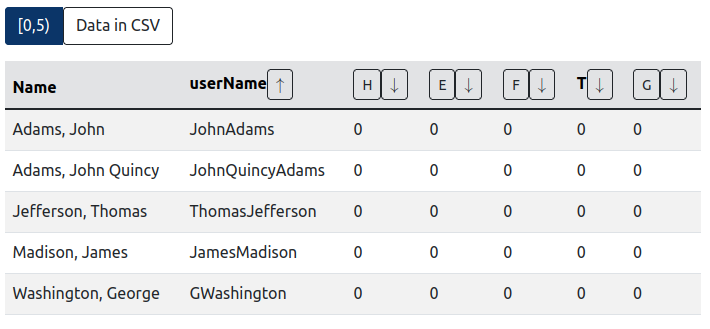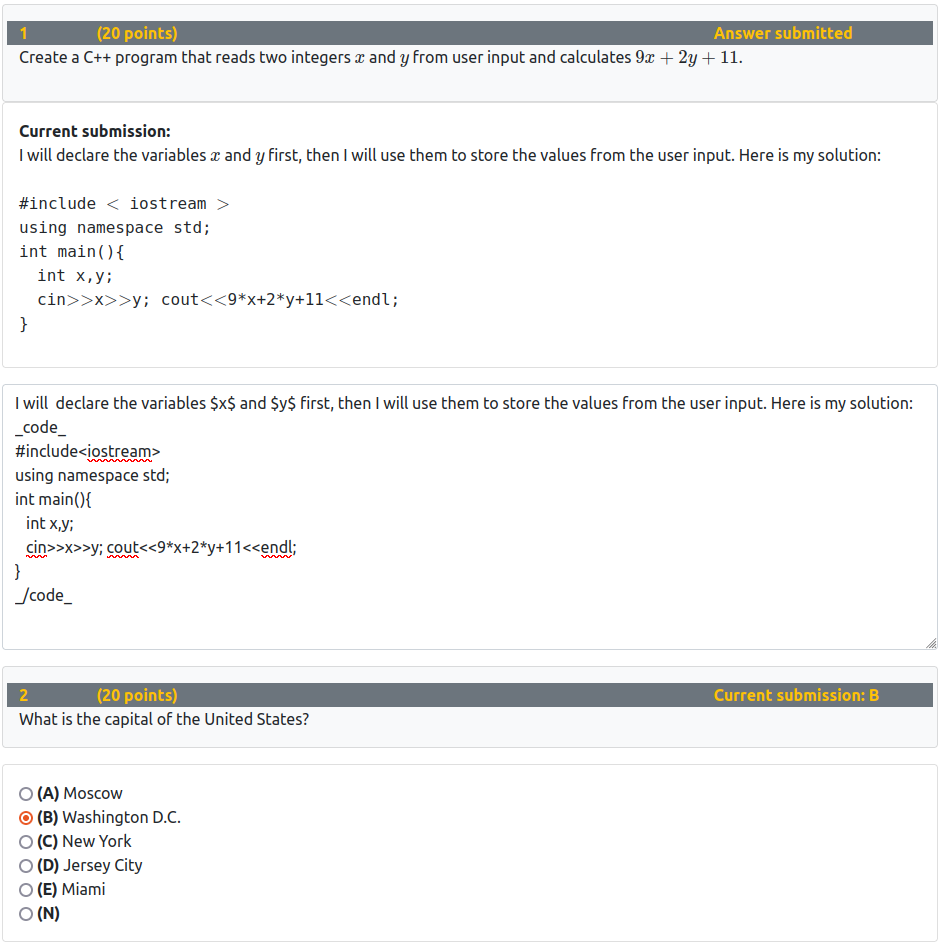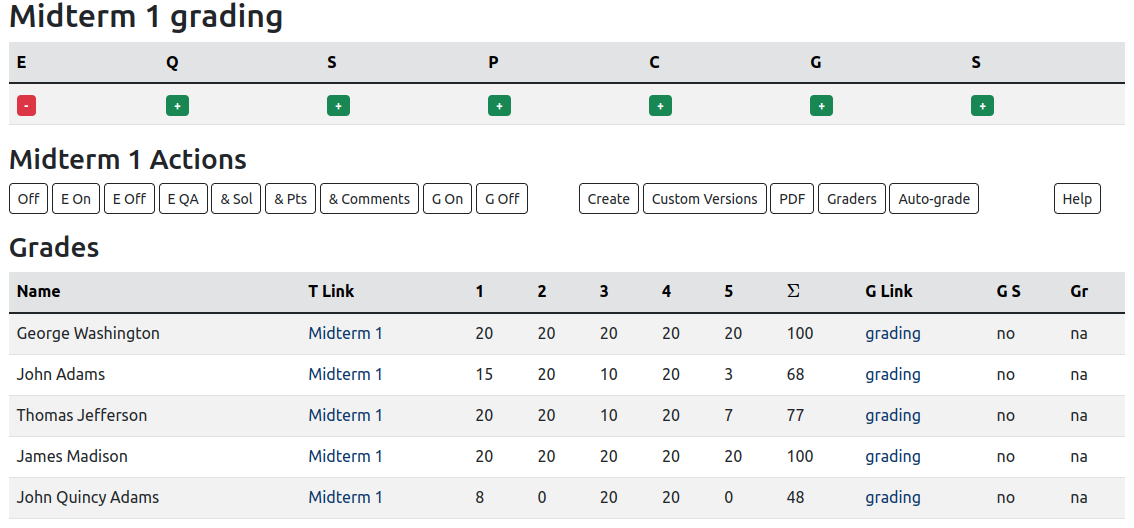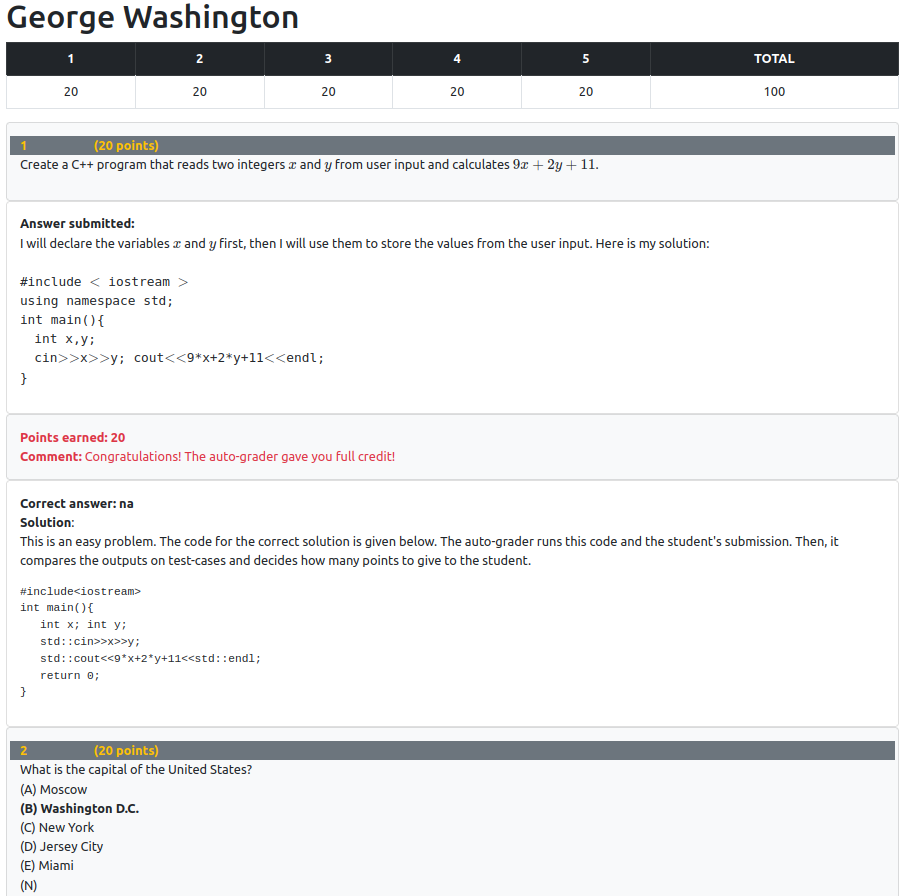The software helps you keep all grades organized. The students will see their grades directly from the website. You will not need to send them grades through emails.

The software supports the creation of questions and exams, distribution of assignments to students, administration of online assignments, and administration of grading jobs.## Exam questions and problems

Each assignment can have a diverse set of problems. The problems can be multiple choice, short answer, programming assignments, or the problems that request essay type answers. The last category is the only one that can't be auto-graded.

The software lets you use random parameters. This makes it easy to give different versions of exam questions to different students. Instead of making the question that asks the students to calculate $$\frac{7+11\cdot 8}5$$, you can replace the numbers $$7$$, $$11$$, $$8$$, and $$5$$ with parameters $$\alpha$$, $$\beta$$, $$\gamma$$, and $$\delta$$. Then you can define the acceptable random values for these parameters and let the program randomize the exams.

## Exam solving